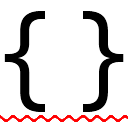#StyleCop.CSharpby StyleCop contributors, Andy Reeves, Chris Dahlberg<PackageReference Include="StyleCop.CSharp" Version="6.2.0" />

# OperatorType

public enum OperatorType
The various operator types.

AndEquals = 19

An AND equals symbol.

A tilde symbol: '~'.

A conditional AND symbol.

A colon: ':'.

A conditional equals symbol: '=='.

A conditional OR symbol: '||'.

A question mark: '?'.

Decrement = 34

A decrement symbol: '--'.

A dereference symbol: '*'.

Division = 25

A division sign: '/'.

A divide equals symbol: '/='.

Equals = 12

An equals sign: '='.

A greater-than sign.

A greater than or equals sign.

Increment = 33

An increment symbol: '++'.

Lambda = 44

The lambda operator: =>

LeftShift = 28

A left-shift symbol.

A left-shift equals sign.

A less-then sign.

A less than or equals sign.

A logical AND symbol.

A logical OR symbol: '|'.

A logical XOR symbol: '^'.

A member access operator: '.'.

Minus = 23

A minus sign: '-'.

A minus equals symbol: '-='.

Mod = 26

A MOD symbol: '%'.

ModEquals = 27

A MOD equals symbol: '%='.

A multiplication sign: '*'.

A times equals symbol: '*='.

Negative = 38

A negative sign: '-'.

Not = 35

A NOT symbol: '!'.

A NOT equals symbol: '!='.

A null coalescing symbol: '??'.

The null conditional operator ?.

OrEquals = 20

An OR equals symbol: '|='.

Plus = 22

A plus sign: '+'.

A plus equals symbol: '+='.

Pointer = 41

A pointer symbol: '->'.

Positive = 37

A positive sign: '+'.

A qualified alias operator: '::'.

A right-shift symbol.

A right-shift equals sign.

XorEquals = 21

An XOR equals symbol: '^='.